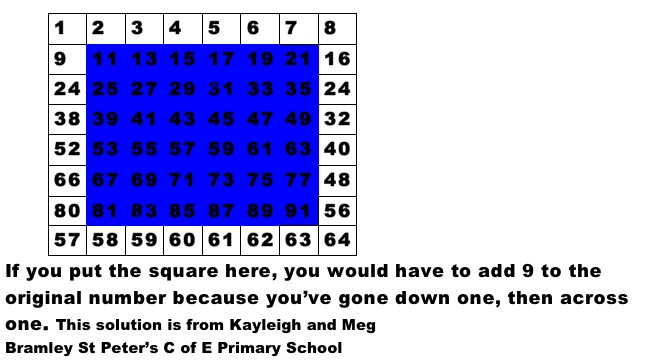#### You may also like### Prompt Cards

These two group activities use mathematical reasoning - one is numerical, one geometric.### Consecutive Numbers

An investigation involving adding and subtracting sets of consecutive numbers. Lots to find out, lots to explore.### Exploring Wild & Wonderful Number Patterns

EWWNP means Exploring Wild and Wonderful Number Patterns Created by Yourself! Investigate what happens if we create number patterns using some simple rules.

# Sorting the Numbers

##### Age 5 to 11Challenge Level

We had some interesting responses from St. James School in Southam. UK.

James, Mathieu, Morgan and Stephen wrote:

On the large square you add $8$ to each column and on the smaller square you add $6$ each time.

Alex, Isaac, Matty and Emil wrote:

$+8$ downwards, $+9$ diagonally right downwards, $+7$ diagonally left downwards

The first box is equivalent to $22$, and then we discovered that if you plussed $8$, then that would be your next boxes answer! Following through right until the end of the larger square!